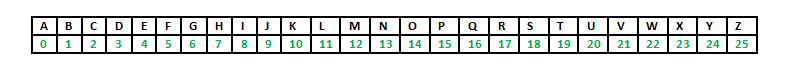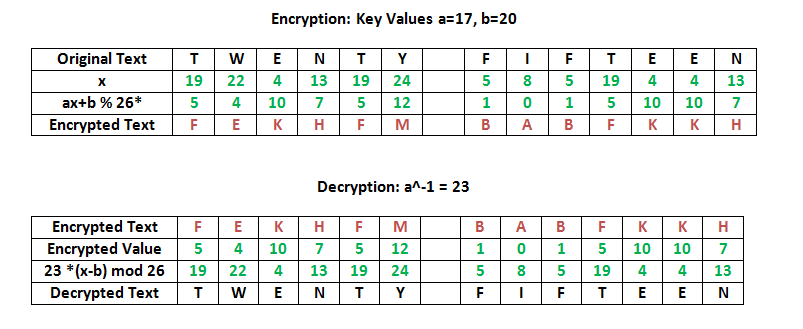Related Articles

# Implementation of Affine Cipher

• Difficulty Level : Expert
• Last Updated : 04 Aug, 2021

The Affine cipher is a type of monoalphabetic substitution cipher, wherein each letter in an alphabet is mapped to its numeric equivalent, encrypted using a simple mathematical function, and converted back to a letter. The formula used means that each letter encrypts to one other letter, and back again, meaning the cipher is essentially a standard substitution cipher with a rule governing which letter goes to which.
The whole process relies on working modulo m (the length of the alphabet used). In the affine cipher, the letters of an alphabet of size m are first mapped to the integers in the range 0 … m-1.

The ‘key’ for the Affine cipher consists of 2 numbers, we’ll call them a and b. The following discussion assumes the use of a 26 character alphabet (m = 26). a should be chosen to be relatively prime to m (i.e. a should have no factors in common with m).Encryption

It uses modular arithmetic to transform the integer that each plaintext letter corresponds to into another integer that correspond to a ciphertext letter. The encryption function for a single letter is

``` E ( x ) = ( a x + b ) mod m
modulus m: size of the alphabet
a and b: key of the cipher.
a must be chosen such that a and m are coprime.```

Decryption

In deciphering the ciphertext, we must perform the opposite (or inverse) functions on the ciphertext to retrieve the plaintext. Once again, the first step is to convert each of the ciphertext letters into their integer values. The decryption function is

```D ( x ) = a^-1 ( x - b ) mod m
a^-1 : modular multiplicative inverse of a modulo m. i.e., it satisfies the equation
1 = a a^-1 mod m .```

To find a multiplicative inverse

We need to find a number x such that:
If we find the number x such that the equation is true, then x is the inverse of a, and we call it a^-1. The easiest way to solve this equation is to search each of the numbers 1 to 25, and see which one satisfies the equation.

```[g,x,d] = gcd(a,m);    % we can ignore g and d, we dont need them
x = mod(x,m);      ```

If you now multiply x and a and reduce the result (mod 26), you will get the answer 1. Remember, this is just the definition of an inverse i.e. if a*x = 1 (mod 26), then x is an inverse of a (and a is an inverse of x)

Example:## C++

 `//CPP program to illustrate Affine Cipher` `#include``using` `namespace` `std;` `//Key values of a and b``const` `int` `a = 17;``const` `int` `b = 20;` `string encryptMessage(string msg)``{``    ``///Cipher Text initially empty``    ``string cipher = ``""``;``    ``for` `(``int` `i = 0; i < msg.length(); i++)``    ``{``        ``// Avoid space to be encrypted``        ``if``(msg[i]!=``' '``)``            ``/* applying encryption formula ( a x + b ) mod m``            ``{here x is msg[i] and m is 26} and added 'A' to``            ``bring it in range of ascii alphabet[ 65-90 | A-Z ] */``            ``cipher = cipher +``                        ``(``char``) ((((a * (msg[i]-``'A'``) ) + b) % 26) + ``'A'``);``        ``else``            ``//else simply append space character``            ``cipher += msg[i];    ``    ``}``    ``return` `cipher;``}` `string decryptCipher(string cipher)``{``    ``string msg = ``""``;``    ``int` `a_inv = 0;``    ``int` `flag = 0;``    ` `    ``//Find a^-1 (the multiplicative inverse of a``        ``//in the group of integers modulo m.)``    ``for` `(``int` `i = 0; i < 26; i++)``    ``{``        ``flag = (a * i) % 26;``        ` `        ``//Check if (a*i)%26 == 1,``                ``//then i will be the multiplicative inverse of a``        ``if` `(flag == 1)``        ``{``            ``a_inv = i;``        ``}``    ``}``    ``for` `(``int` `i = 0; i < cipher.length(); i++)``    ``{``        ``if``(cipher[i]!=``' '``)``            ``/*Applying decryption formula a^-1 ( x - b ) mod m``            ``{here x is cipher[i] and m is 26} and added 'A'``            ``to bring it in range of ASCII alphabet[ 65-90 | A-Z ] */``            ``msg = msg +``                       ``(``char``) (((a_inv * ((cipher[i]+``'A'` `- b)) % 26)) + ``'A'``);``        ``else``            ``//else simply append space character``            ``msg += cipher[i];``    ``}` `    ``return` `msg;``}` `//Driver Program``int` `main(``void``)``{``    ``string msg = ``"AFFINE CIPHER"``;``    ` `    ``//Calling encryption function``    ``string cipherText = encryptMessage(msg);``    ``cout << ``"Encrypted Message is : "` `<< cipherText<

## Java

 `// Java program to illustrate Affine Cipher` `class` `GFG``{` `    ``// Key values of a and b``    ``static` `int` `a = ``17``;``    ``static` `int` `b = ``20``;` `    ``static` `String encryptMessage(``char``[] msg)``    ``{``        ``/// Cipher Text initially empty``        ``String cipher = ``""``;``        ``for` `(``int` `i = ``0``; i < msg.length; i++)``        ``{``            ``// Avoid space to be encrypted``            ``/* applying encryption formula ( a x + b ) mod m``            ``{here x is msg[i] and m is 26} and added 'A' to``            ``bring it in range of ascii alphabet[ 65-90 | A-Z ] */``            ``if` `(msg[i] != ``' '``)``            ``{``                ``cipher = cipher``                        ``+ (``char``) ((((a * (msg[i] - ``'A'``)) + b) % ``26``) + ``'A'``);``            ``} ``else` `// else simply append space character``            ``{``                ``cipher += msg[i];``            ``}``        ``}``        ``return` `cipher;``    ``}` `    ``static` `String decryptCipher(String cipher)``    ``{``        ``String msg = ``""``;``        ``int` `a_inv = ``0``;``        ``int` `flag = ``0``;` `        ``//Find a^-1 (the multiplicative inverse of a``        ``//in the group of integers modulo m.)``        ``for` `(``int` `i = ``0``; i < ``26``; i++)``        ``{``            ``flag = (a * i) % ``26``;` `            ``// Check if (a*i)%26 == 1,``            ``// then i will be the multiplicative inverse of a``            ``if` `(flag == ``1``)``            ``{``                ``a_inv = i;``            ``}``        ``}``        ``for` `(``int` `i = ``0``; i < cipher.length(); i++)``        ``{``            ``/*Applying decryption formula a^-1 ( x - b ) mod m``            ``{here x is cipher[i] and m is 26} and added 'A'``            ``to bring it in range of ASCII alphabet[ 65-90 | A-Z ] */``            ``if` `(cipher.charAt(i) != ``' '``)``            ``{``                ``msg = msg + (``char``) (((a_inv *``                        ``((cipher.charAt(i) + ``'A'` `- b)) % ``26``)) + ``'A'``);``            ``}``            ``else` `//else simply append space character``            ``{``                ``msg += cipher.charAt(i);``            ``}``        ``}` `        ``return` `msg;``    ``}` `    ``// Driver code``    ``public` `static` `void` `main(String[] args)``    ``{``        ``String msg = ``"AFFINE CIPHER"``;` `        ``// Calling encryption function``        ``String cipherText = encryptMessage(msg.toCharArray());``        ``System.out.println(``"Encrypted Message is : "` `+ cipherText);` `        ``// Calling Decryption function``        ``System.out.println(``"Decrypted Message is: "` `+ decryptCipher(cipherText));` `    ``}``}` `// This code contributed by Rajput-Ji`

## Python

 `# Implementation of Affine Cipher in Python` `# Extended Euclidean Algorithm for finding modular inverse``# eg: modinv(7, 26) = 15``def` `egcd(a, b):``    ``x,y, u,v ``=` `0``,``1``, ``1``,``0``    ``while` `a !``=` `0``:``        ``q, r ``=` `b``/``/``a, b``%``a``        ``m, n ``=` `x``-``u``*``q, y``-``v``*``q``        ``b,a, x,y, u,v ``=` `a,r, u,v, m,n``    ``gcd ``=` `b``    ``return` `gcd, x, y` `def` `modinv(a, m):``    ``gcd, x, y ``=` `egcd(a, m)``    ``if` `gcd !``=` `1``:``        ``return` `None`  `# modular inverse does not exist``    ``else``:``        ``return` `x ``%` `m`  `# affine cipher encryption function``# returns the cipher text``def` `affine_encrypt(text, key):``    ``'''``    ``C = (a*P + b) % 26``    ``'''``    ``return` `'``'.join([ chr((( key*(ord(t) - ord('``A')) ``+` `key[``1``] ) ``%` `26``)``                  ``+` `ord``(``'A'``)) ``for` `t ``in` `text.upper().replace(``' '``, '') ])`  `# affine cipher decryption function``# returns original text``def` `affine_decrypt(cipher, key):``    ``'''``    ``P = (a^-1 * (C - b)) % 26``    ``'''``    ``return` `'``'.join([ chr((( modinv(key, 26)*(ord(c) - ord('``A') ``-` `key[``1``]))``                    ``%` `26``) ``+` `ord``(``'A'``)) ``for` `c ``in` `cipher ])`  `# Driver Code to test the above functions``def` `main():``    ``# declaring text and key``    ``text ``=` `'AFFINE CIPHER'``    ``key ``=` `[``17``, ``20``]` `    ``# calling encryption function``    ``affine_encrypted_text ``=` `affine_encrypt(text, key)` `    ``print``(``'Encrypted Text: {}'``.``format``( affine_encrypted_text ))` `    ``# calling decryption function``    ``print``(``'Decrypted Text: {}'``.``format``    ``( affine_decrypt(affine_encrypted_text, key) ))`  `if` `__name__ ``=``=` `'__main__'``:``    ``main()``# This code is contributed by``# Bhushan Borole`

## C#

 `// C# program to illustrate Affine Cipher``using` `System;``    ` `class` `GFG``{` `    ``// Key values of a and b``    ``static` `int` `a = 17;``    ``static` `int` `b = 20;` `    ``static` `String encryptMessage(``char``[] msg)``    ``{``        ``/// Cipher Text initially empty``        ``String cipher = ``""``;``        ``for` `(``int` `i = 0; i < msg.Length; i++)``        ``{``            ``// Avoid space to be encrypted``            ``/* applying encryption formula ( a x + b ) mod m``            ``{here x is msg[i] and m is 26} and added 'A' to``            ``bring it in range of ascii alphabet[ 65-90 | A-Z ] */``            ``if` `(msg[i] != ``' '``)``            ``{``                ``cipher = cipher``                        ``+ (``char``) ((((a * (msg[i] - ``'A'``)) + b) % 26) + ``'A'``);``            ``} ``else` `// else simply append space character``            ``{``                ``cipher += msg[i];``            ``}``        ``}``        ``return` `cipher;``    ``}` `    ``static` `String decryptCipher(String cipher)``    ``{``        ``String msg = ``""``;``        ``int` `a_inv = 0;``        ``int` `flag = 0;` `        ``//Find a^-1 (the multiplicative inverse of a``        ``//in the group of integers modulo m.)``        ``for` `(``int` `i = 0; i < 26; i++)``        ``{``            ``flag = (a * i) % 26;` `            ``// Check if (a*i)%26 == 1,``            ``// then i will be the multiplicative inverse of a``            ``if` `(flag == 1)``            ``{``                ``a_inv = i;``            ``}``        ``}``        ``for` `(``int` `i = 0; i < cipher.Length; i++)``        ``{``            ``/*Applying decryption formula a^-1 ( x - b ) mod m``            ``{here x is cipher[i] and m is 26} and added 'A'``            ``to bring it in range of ASCII alphabet[ 65-90 | A-Z ] */``            ``if` `(cipher[i] != ``' '``)``            ``{``                ``msg = msg + (``char``) (((a_inv *``                        ``((cipher[i] + ``'A'` `- b)) % 26)) + ``'A'``);``            ``}``            ``else` `//else simply append space character``            ``{``                ``msg += cipher[i];``            ``}``        ``}` `        ``return` `msg;``    ``}` `    ``// Driver code``    ``public` `static` `void` `Main(String[] args)``    ``{``        ``String msg = ``"AFFINE CIPHER"``;` `        ``// Calling encryption function``        ``String cipherText = encryptMessage(msg.ToCharArray());``        ``Console.WriteLine(``"Encrypted Message is : "` `+ cipherText);` `        ``// Calling Decryption function``        ``Console.WriteLine(``"Decrypted Message is: "` `+ decryptCipher(cipherText));` `    ``}``}` `/* This code contributed by PrinciRaj1992 */`

Output:

```Encrypted Message is : UBBAHK CAPJKX
Decrypted Message is : AFFINE CIPHER```

This article is contributed by Yasin Zafar. If you like GeeksforGeeks and would like to contribute, you can also write an article using write.geeksforgeeks.org or mail your article to review-team@geeksforgeeks.org. See your article appearing on the GeeksforGeeks main page and help other Geeks.